9

Bitwise operations

There is hardly anything that is unconditional in the world today. To cater to this real world situation, the WMLScript language has also provided conditional statements. These encompass the conditional AND, OR and Exclusive OR statements. They enable you to conditionally execute certain statements in the program. This chapter shall explain the mysteries of these operators and their utility in programs. The chapter shall also familiarize you with the low-level bit-wise Right Shift and Left Shift operators. These enable you to do bit-wise manipulations.

The AND Conditional Statement

The two ampersands && represent the logical AND condition.

aaa.wmls

extern function abc()

{

var aa,i;

i = 10;

for ( i=1;i<=5;i++)

{

if (i >=2 && i <=4 )

{

aa = String.format("%d..",i);

}

}

}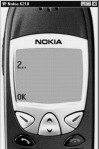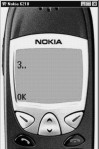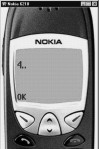Screen 9. 1 Screen 9. 2 Screen 9. 3

AND represents a logical condition which will return a true only when both the conditions, i.e. the ones preceding and following the AND are true. In the context of the above example, AND implies that only when both the conditions are true, the if statement is said to be true. In the loop, the value of i ranges from 1 to 5, but the if statement stands true only for values 2,3,4. And so, you will realize that the String.format and the Dialogs.alert are executed only when the value of i is 2,3 or 4.  The AND condition makes the if more restrictive and also renders it more intelligent. This is so because in such a case we can specify that unless both the conditions are true, the if statement will not be true. It is pertinent to note that you can have as many AND conditions as you want. Writing of intelligent programs precludes the usage of such constructs.

The OR Conditional Statement

The OR conditional statement is represented by two pipe symbols i.e. ||. Where the AND makes a statement more restrictive, the OR condition makes it less restrictive.  In the above example, you can't replace the && with the || condition because it will not make any logical sense.

aaa.wmls

extern function abc()

{

var aa,i;

i = 10;

for ( i=1;i<=5;i++)

{

if (i <=2 || i >=4 )

{

aa = String.format("%d..",i);

}

}

}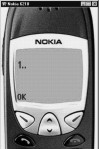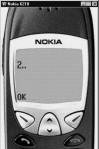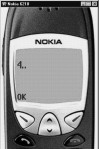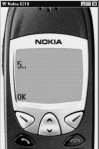Screen 9. 4 Screen 9. 5 Screen 9. 6 Screen 9. 7

Depending upon the situation, you will either need to use the AND or the OR conditional statement. Both are indispensable in their respective contexts. In real world programming, both the AND and the OR statements will be required under different circumstances. One cannot be replaced with the other.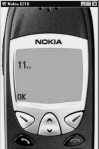Screen 9. 8

aaa.wmls

extern function abc()

{

var aa,i;

i = 10;

if ( true && ++i)

;

aa = String.format("%d..",i);

}

There is a very basic philosophy regarding the C programming language and any other language that has been derived from C. According to this philosophy, a function or an operator or a program statement should not go beyond what is necessary; however, if it does something extra, it should not be infested with undesirable side effects.  What we mean is that the answer should not get modified due to this.

Now, let us look at the if statement. The if statement has an AND . The AND condition implies that both the conditions should be true. If one of the conditions is false, then if is considered false. The if statement consists of a "true" which will always be true. It also contains the statement ++i. The first condition will always be true, but there is a possibility that the second one could be false. If so, then the result of the AND condition will change. So, C or WMLScript have no choice but to execute the statement ++i to evaluate whether it is true or false. Here, since the value of i becomes 11 ( which is a number greater than 0), second condition is also true. So, the value of i in the Dialogs.alert will be 11.

So, in an AND condition, if the first condition is executed and it returns a value of true, then the second statement gets executed.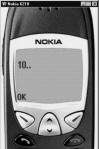Screen 9. 9

aaa.wmls

extern function abc()

{

var aa,i;

i = 10;

if ( false && ++i)

;

aa = String.format("%d..",i);

}

In the above example, the first condition of the if statement is false. Under these circumstance, whether the second condition returns a true or false, it will have no bearing on the final return value of the if statement, which is false. So, the second condition does not get executed because the first one is already false. Hence, the value of i will remain 10.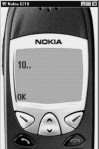Screen 9. 10

aaa.wmls

extern function abc()

{

var aa,i;

i = 10;

if ( true ||  ++i)

;

aa = String.format("%d..",i);

}

In the above example, we have used if true in the OR statement. Here, since the first condition is true, the program will not execute the second condition. This is because, even if it executes the second statement, the final result of the if statement will remain exactly the same. The OR statement just requires one of the conditions to be true, in order to return a value of true. Here, since the first condition is true, the final value of the if statement remains unaffected, even though the second one may result in a true or a false value. The if statement will return a value of true on account of the first condition itself. Hence, the value of i will remain 10.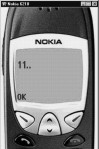Screen 9. 11

aaa.wmls

extern function abc()

{

var aa,i;

i = 10;

if ( false ||  ++i)

;

aa = String.format("%d..",i);

}

Here, though the first condition is false, the if statement can still hold true if the next condition is true. So, there is a valid reason for the next condition to be executed. So, the output of the program will be 11.

These are very basic concepts. If you are unable to comprehend them clearly, you will find yourself in a tricky situation where you  will not be able to decipher as to why your code is not working.

· The two ampersand symbols && represent the AND condition.

· The double pipe symbols || represent the OR condition.

Bitwise operations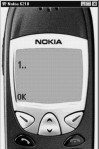Screen 9. 12

aaa.wmls

extern function abc()

{

var aa,i;

i = 3 & 5;

aa = String.format("%d..",i);

}

In a computer's memory, you have a byte.  A byte is made up of 8 bits ( in our case, 8 boxes). We start counting from 0. So, we number the boxes from 0 upto 7, and we give each one of them a weightage of the order of 2. So, if all the bits contain a 0, the memory location will contain the value 0.

 7 6 5 4 3 2 1 0 0 0 0 0 0 0 0 0 128 64 32 16 8 4 2 1

if it contains 1,

 7 6 5 4 3 2 1 0 0 0 0 0 0 0 0 1 128 64 32 16 8 4 2 1

it contains the value of 100.

 7 6 5 4 3 2 1 0 0 1 1 0 0 1 0 0 128 64 32 16 8 4 2 1

Now, if you want to represent 3 and 5, this is how you can represent them :

3

 7 6 5 4 3 2 1 0 0 0 0 0 0 0 1 1 128 64 32 16 8 4 2 1

5

 7 6 5 4 3 2 1 0 0 0 0 0 0 1 0 1 128 64 32 16 8 4 2 1

The first two bits have the value of 1 for number 3. The first and third bits have the value 1 for the number 5.  For a bitwise AND condition to return true, both the conditions have to be true. However, If either one of them is false, it will return false. In this case, since both the bits have a value of 1in the first box, the answer will be 1.

1

 7 6 5 4 3 2 1 0 0 0 0 0 0 0 0 1 128 64 32 16 8 4 2 1

You may wonder as to where bitwise AND operator can be used. If you indulge in cryptography, you will realise that bitwise AND operator is indispensable. Secondly, you can use it to access a specific memory location. If you want to turn a certain bit off, then you can "bitwise AND" it with a value of 0.  When you bitwise AND with a 0, you are ensuring that the resultant value of the bit remains 0, irrespective of the original value of the bit. If you bitwise AND with a value of 1, the value of the bit remains the same, just like its original value.

Eg. For an original value of 0, 0 && 1 will give a resultant value of 0.

For an original value of 1, 1 && 1 will give a resultant value of 1.

· The single ampersand symbol & represents the bitwise AND operator.

· The single pipe symbol | represents the bitwise OR operator.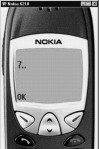Screen 9. 13

aaa.wmls

extern function abc()

{

var aa,i;

i = 3 | 5;

aa = String.format("%d..",i);

}

Now, let us consider the bitwise OR operator. Here, if any of the bits has the value of 1, the result of the ORing will also be 1.

3

 7 6 5 4 3 2 1 0 0 0 0 0 0 0 1 1 128 64 32 16 8 4 2 1

5

 7 6 5 4 3 2 1 0 0 0 0 0 0 1 0 1 128 64 32 16 8 4 2 1

7

 7 6 5 4 3 2 1 0 0 0 0 0 0 1 1 1 128 64 32 16 8 4 2 1

If you want the bit to retain its original value, you have to bitwise OR it with the value 0.

· 1 | 0 gives you a resultant value of 1

· 0 | 0 also gives you a resultant value of 0.

So, if you bitwise OR with a 0, you will get the original value, as the result.

If you bitwise OR with a 1, you will always get a resultant value of 1.

So, whenever you want to turn a bit on, you can bitwise OR it with 1, and when you want to turn it off, you bitwise AND it with 0.

Now, we shall delve upon the mystery of the Exclusive OR. It is represented by the carat sign i.e. ^.

In exclusive ORing, the thumb rule is that, only if we have the expression 0^1 or 1^0 , the answer will be 1. In all other cases including 0^0 and 1^1, the answer will be 0. This is the only deviation from the bitwise OR condition.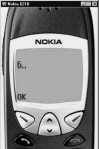Screen 9. 14

aaa.wmls

extern function abc()

{

var aa,i;

i = 3 ^ 5;

aa = String.format("%d..",i);

}

When you use bitwise Exclusive OR, you get a 6 and not 7. This happens because the first bits each, of both these numbers have the value of 1, and  1^1 will give you a resultant value of 0.

3

 7 6 5 4 3 2 1 0 0 0 0 0 0 0 1 1 128 64 32 16 8 4 2 1

5

 7 6 5 4 3 2 1 0 0 0 0 0 0 1 0 1 128 64 32 16 8 4 2 1

6

 7 6 5 4 3 2 1 0 0 0 0 0 0 1 1 0 128 64 32 16 8 4 2 1

If bitwise Exclusive OR the number 6 with 3, you will get 5.

If bitwise Exclusive OR the number 6 with 5, you will get 3.

6

 7 6 5 4 3 2 1 0 0 0 0 0 0 1 1 0 128 64 32 16 8 4 2 1

3

 7 6 5 4 3 2 1 0 0 0 0 0 0 1 1 0 128 64 32 16 8 4 2 1

6^3=5

5

 7 6 5 4 3 2 1 0 0 0 0 0 0 1 0 1 128 64 32 16 8 4 2 1

This can be used for a rudimentary cryptography illustration, where you take every byte from the file and, bitwise Exclusive OR it with a certain number. You can store the resultant number and thereafter, pass that number to the person who receives your encrypted message. When that person uses bitwise Exclusive ORing on the encrypted message using the same number, he will get back the original message. This is what Exclusive ORing is meant for.

The two >> signs represent the operation of right shifting.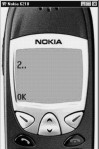Screen 9. 15

aaa.wmls

extern function abc()

{

var aa,i;

i = 8 >> 2;

aa = String.format("%d..",i);

}

Right shift  denotes that you are taking all the bits and shifting them to the right. When you right shift by 2, the bytes shift to the right by 2 positions each, such that the two leftmost bits now get replaced with 0.

So, when you right shift, it is equivalent to multiplying the number by 2 for every position shifted. And when you left shift, the bits get shifted to the left, which is similar to multiplying the number by 2 for every position that the bits are shifted. .

8

 7 6 5 4 3 2 1 0 0 0 0 0 1 0 0 0 128 64 32 16 8 4 2 1

8 >> 2=2

 7 6 5 4 3 2 1 0 0 0 0 0 0 0 1 0 128 64 32 16 8 4 2 1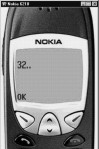Screen 9. 16

aaa.wmls

extern function abc()

{

var aa,i;

i = 8 << 2;

aa = String.format("%d..",i);

}

8

 7 6 5 4 3 2 1 0 0 0 0 0 1 0 0 0 128 64 32 16 8 4 2 1

8<<2 = 32

 7 6 5 4 3 2 1 0 0 0 1 0 0 0 0 0 128 64 32 16 8 4 2 1